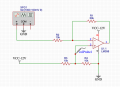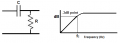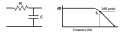# Basic OpAmp question

#### doubledutch1962

Joined Oct 27, 2020
37
I would appreciate it if someone could give me some guidance. I appreciate that the below circuit is simple and doesn't contain some of the capacitors but I tried adding those as well and it seems to make no difference with what I am experiencing. When I attach a scope to the output, pin 1 of this LM358 OpAmp, the signal is a straight line clamped against the top rail.

However, I do get the amplified Sine Wave when I change resistors R3 and R4 so the voltage at volProve1 = 1V (and not 6 Volts) and I don't understand why that is. I thought the R3/R4 voltage divider raises the virtual ground by 6 Volts so the amplified signal is offset against that 6V virtual ground but that doesn't seem to happen. Apologies if this is a very basic question.#### LesJones

Joined Jan 8, 2017
3,748
The first problem is VCC should be a positive voltage not a negative voltage.
If you correct the supply voltage polarity problem you would still have a problem.
As pin 3 would be biased to + 6 volts the op amp will attempt to get pin 2 to also be at + 6 volts. As the DC level on the left hand end of R2 will be 0 volts (Assuming the output of the signal generator is directly coupled.) the op amp output would have to be at +36 volts to get + 6 volts on pin 2 ( 6 v x (10000 + 50000)/1000). As the supply voltage is + 12 volts the output can never reach +36 volts. (36 volts would also exceed the maximum output voltage of the device.) Connecting a capacitor in series with R2 would solve this problem.

Les.

#### crutschow

Joined Mar 14, 2008
30,116
If you calculate the voltages at the two op amp inputs you will see the problem.
The two inputs have be the same voltage (within a few microvolts) for the opamp to properly work.
If not, then then op amp output will be at one of the rails.

The voltages and resistor values you show, don't allow for that.
If pin 3 is at 6V, how can pin 2 also get to 6V?

#### MrChips

Joined Oct 2, 2009
26,504
Op-amp basics

When negative feedback is correctly applied and functioning, the inverting and non-inverting inputs must be at the same voltage otherwise the output will hit the rail. Remember that the gain of an ideal op-amp is infinite.

#### Audioguru again

Joined Oct 21, 2019
4,798
Your schematic was missing a few important parts. Do it like this:

#### doubledutch1962

Joined Oct 27, 2020
37
Apologies for the delay, and thank you all for your very helpful suggestions. With the combined posts above, I believe I know what's going on now.

#### doubledutch1962

Joined Oct 27, 2020
37
Can I ask a follow-up question, based on the recommended circuit suggested by @Audioguru again? Why did he suggest the capacitors he did of 1uF on the input, and 100uF and 3.3uF close to the voltage divider?

They work perfectly but I'm trying to figure out what the theory is for having selected these values based on the circuit I provided. Could someone point me in the right direction so I can learn about this and don't have to ask next time?

#### MrChips

Joined Oct 2, 2009
26,504
Can I ask a follow-up question, based on the recommended circuit suggested by @Audioguru again? Why did he suggest the capacitors he did of 1uF on the input, and 100uF and 3.3uF close to the voltage divider?

They work perfectly but I'm trying to figure out what the theory is for having selected these values based on the circuit I provided. Could someone point me in the right direction so I can learn about this and don't have to ask next time?
Resistors and capacitors (and every circuit element) perform a specific function.
You need to identify what function that component plays in the circuit.

Capacitors are very often used in filter circuits, low pass and high pass filters.
An RC filter has a cut-off frequency given by:

fc = 1 / (2πRC)

Hence first determine if you need to implement a low pass filter or a high pass filter. Next, determine a desirable cut-off frequency.
Once you have chosen a desired cut-off frequency you can select R and C values to achieve your objectives.

#### LesJones

Joined Jan 8, 2017
3,748
The 1 uF capacitor on the input just block the DC path. I just needs to have a low reactance with respect to R2 (10K) I am assuming the signal frequency is 1000 hz. If this assumption is correct then 1 uf will have a reactance of about 160 ohms so it will only cause a reduction of the signal by about 1.6%.
The 100 uF in parallel with the power supply is to help reduce any noise from the power supply and helps to reduce the source impedance of the power supply at the signal frequency.
The 3,3uF capacitor provides filtering to reduce any noise pickup on the reference voltage on pin 3 of the op amp.

Les.

•doubledutch1962

#### Audioguru again

Joined Oct 21, 2019
4,798
Audio has frequencies from 20Hz to 20kHz. Bass sounds are from 20Hz to about 200Hz.
The formula for a capacitor that feeds a resistor is 1/( 2 x pi x R x frequency).
My calculation for the input capacitor is 1/(2 x 3.142 x 10k x 20Hz)= 0.7uF for a loudness reduction of -3dB but I selected the next higher capacitance so that 20Hz would not be reduced much.

I selected 3.3uF as a filter capacitor so that electrical 50Hz or 60Hz is reduced but the capacitor can charge fairly quickly. The two 10k resistors are effectively 5k ohms but then the filtering is small at 61Hz and the charging time is too quick using 3.3uF. I should have used 33uF or more.

I selected 100uF parallel to the battery or power supply because its impedance at low frequencies is low and it also works well at high audio frequencies.

#### doubledutch1962

Joined Oct 27, 2020
37

#### MrChips

Joined Oct 2, 2009
26,504
In a nutshell, if the capacitor is in series, it is a high-pass filter. It removes DC and low frequencies.If the capacitor is in parallel, it is a low-pass filter. It limits the upper end of the frequency spectrum and removes high frequency noise.